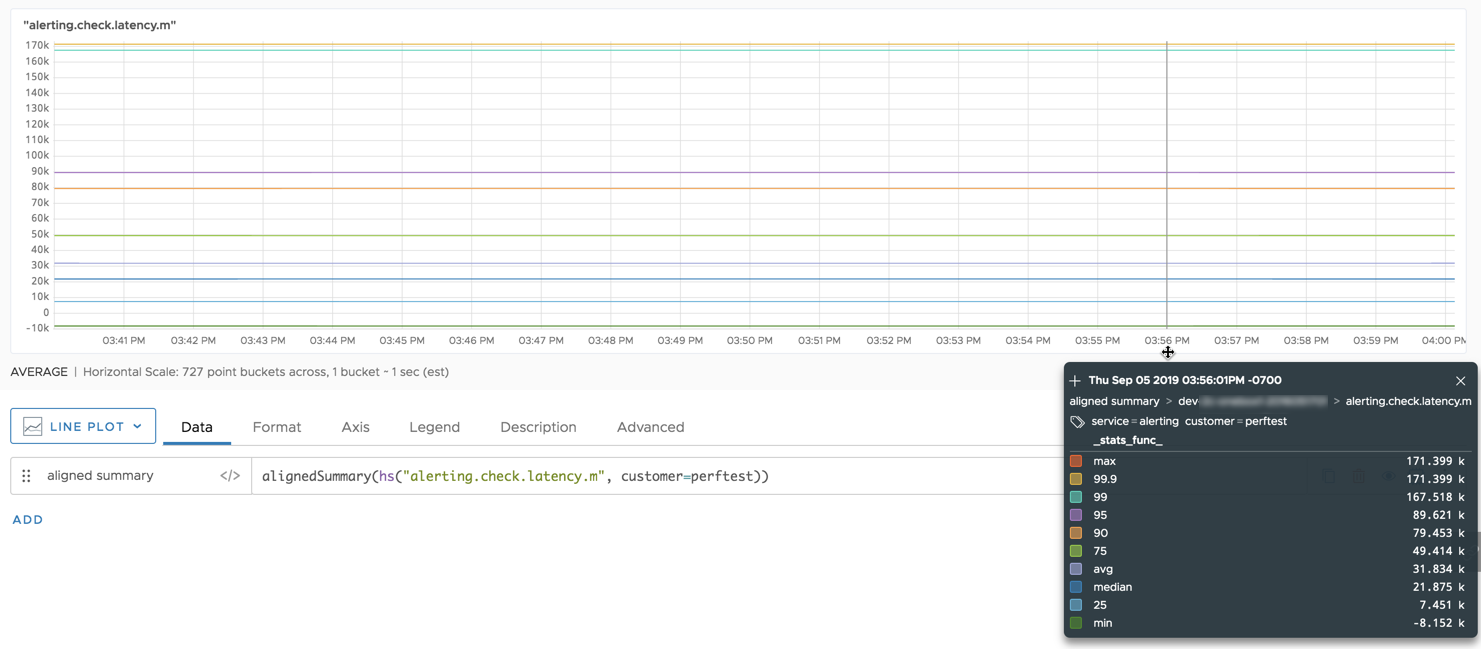Reference to the alignedSummary() function

## Summary

``````alignedSummary(<percentageList>, <hsExpression>)
``````

Aligns the distributions of a histogram series into a single time bucket for the current chart (1vw), and then returns the significant values from the resulting composite distribution. The summary includes a constant time series for each `percentage` percentile.

## Parameters

ParameterDescription
percentageList Comma-separated list of percentages that specify the percentiles you want in your aligned summary. A percentile is the value below which a given percentage of values fall in a histogram distribution. Specify:
• A number from 0 to 100, inclusive. May include a decimal point.
• `max` (equivalent to 100)
• `median` (equivalent to 50)
• `min` (equivalent to 0)
• `avg` (average of the distribution’s values)
You can omit the percentageList to return the default summary, which includes max, 99.9, 99, 95, 90, 75, avg, median (50), 25, and min.
hsExpression Expression describing the histogram series to align and summarize.

## Description

The `alignedSummary()` histogram function gives an overall picture of the histogram distributions in a chart’s time window. For each histogram series described by the expression, `alignedSummary()`:

1. Aligns the distributions across the current chart window to produce a single composite distribution.
2. Summarizes the significant values from the composite distribution.
3. Extends the summary across the chart as constant time series.

The following two queries are therefore equivalent:

``````alignedsummary(hs("my.metric.m))

last(summary(align(1vw, (hs("my.metric.m)))))
``````

The aligned summary for a histogram series returns a constant time series for each requested percentile. A percentile is a value below which a particular percentage of values fall. For example, `alignedSummary(75, 99.99, hs(my.hsMetric.m))` returns 2 constant time series: one for the value at the 75th percentile and one for the value at the 99.99th percentile from the composite distribution.

By default, `alignedSummary()` returns a constant time series for each the following: max, 99.9, 99, 95, 90, 75, avg, median (50), 25, and min.

## Examples

Suppose you want to see the default percentiles for all the histogram distributions that fall between 3:40pm and 4:00pm. You adjust your chart to display that time window, and then run the query `alignedSummary(hs("alerting.check.latency.m", customer=perftest))`. The query returns 10 constant series, one for each of the default percentiles.